Browse by Topic

# Classifying Cough Sounds to Predict COVID-19 Diagnosis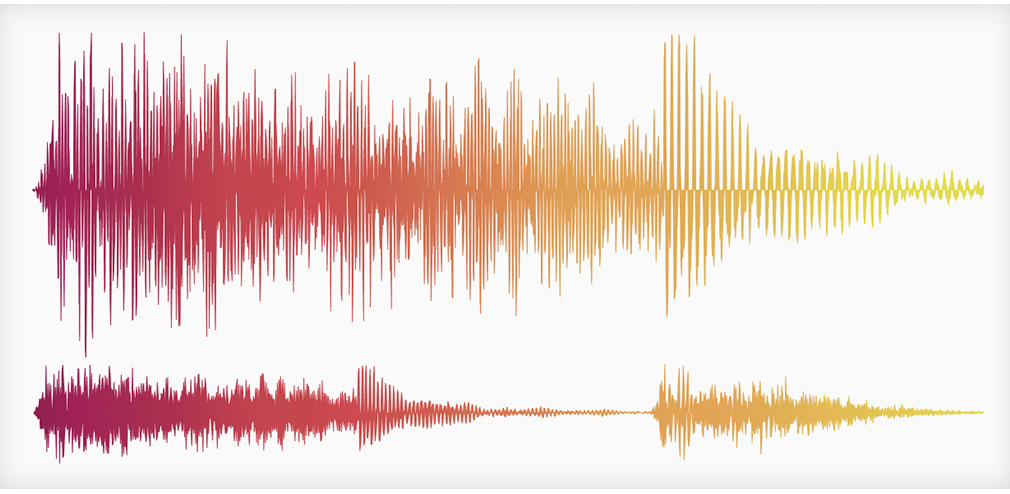Sound classification can be a hard task, especially when sound samples have small variations that can be imperceptible to the human ear. The use of machines, and recently machine learning models, has been shown to be an effective approach to solving the problem of classifying sounds. These applications can help improve diagnoses and have been a topic of research in areas such as cardiology and pulmonology. Recent innovations such as a convolutional neural network identifying COVID-19 coughs and the MIT AI model detecting asymptomatic COVID-19 infections using cough recordings show some promising results for identifying COVID-19 patients just by the sound of their coughs. Looking at these references, this task may look quite challenging and like something that can be done only by top-notch researchers. In this post, we will discuss how you can get very promising results using the machine learning and audio functionalities in the Wolfram Language.

Using a labeled COVID-19 open-source cough-sound dataset, we construct a recurrent neural network and feed in preprocessed audio signals using Mel-frequency cepstral coefficient (MFCC) feature extraction. This approach gave us an accuracy of around 96%, which is similar to the results obtained in different published studies, even when our data was limited to only 121 samples.

The data we used consists of 121 segmented samples of cough sounds in .mp3 format, which are publicly available here. This data has two classes: 48 samples from patients who tested positive for COVID-19 and 73 samples from patients who tested negative for COVID-19: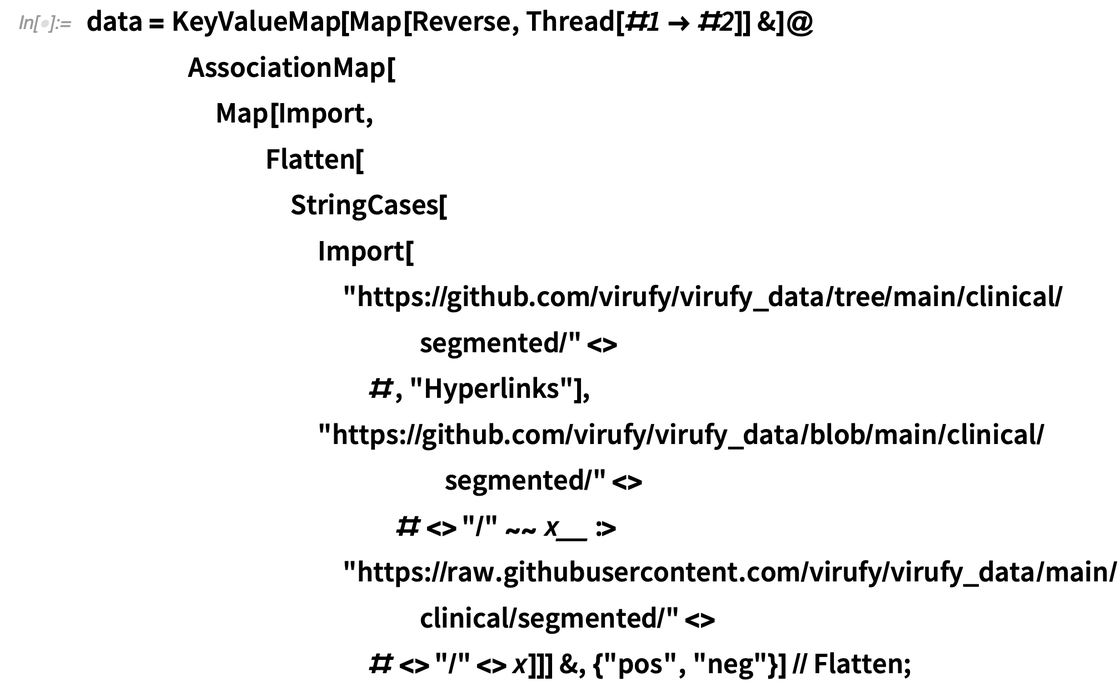✕ ```data = KeyValueMap[Map[Reverse, Thread[#1 -> #2]] &]@ AssociationMap[ Map[Import, Flatten[StringCases[ Import["https://github.com/virufy/virufy_data/tree/main/\ clinical/segmented/" <> #, "Hyperlinks"], "https://github.com/virufy/virufy_data/blob/main/clinical/\ segmented/" <> # <> "/" ~~ x__ :> "https://raw.githubusercontent.com/virufy/virufy_data/main/\ clinical/segmented/" <> # <> "/" <> x]]] &, {"pos", "neg"}] // Flatten;```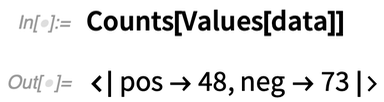✕ `Counts[Values[data]]`

For example, these are random samples from each of the classes in the data: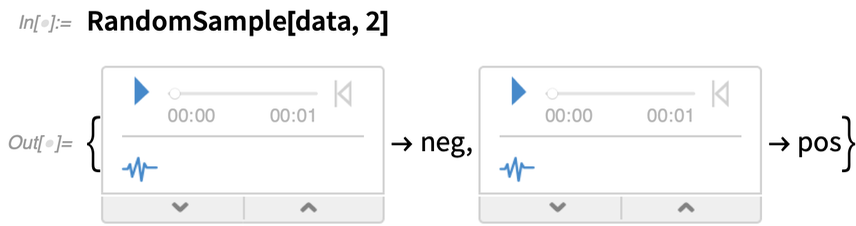✕ `RandomSample[data, 2]`

Although there is an imbalance in sample sizes, the difference is small enough that the model should still be effective. We use TrainTestSplit from the Wolfram Function Repository to create the training and test sets. By default, it will split the data into 80% training and 20% test: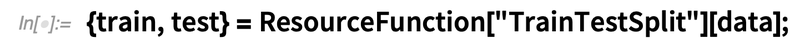✕ `{train, test} = ResourceFunction["TrainTestSplit"][data];`

Audio encoding is an important step for audio classification, as any sound generated by humans is determined by the shape of their vocal tracts (including tongue, teeth, etc.). If this shape can be determined correctly, any sound produced can be accurately represented. The same happens with musical instruments: even when two different instruments can generate the same sound frequency, they will sound different because of the physical characteristics of the instrument (piano, guitar, flute, etc.). The envelope of the time power spectrum of the speech signal is representative of the vocal tract, which MFCCs accurately represent. Some diseases, such as a pulmonary disease, can affect the way the air travels through our respiratory system, so it may cause a difference in sound between a healthy patient and a sick patient: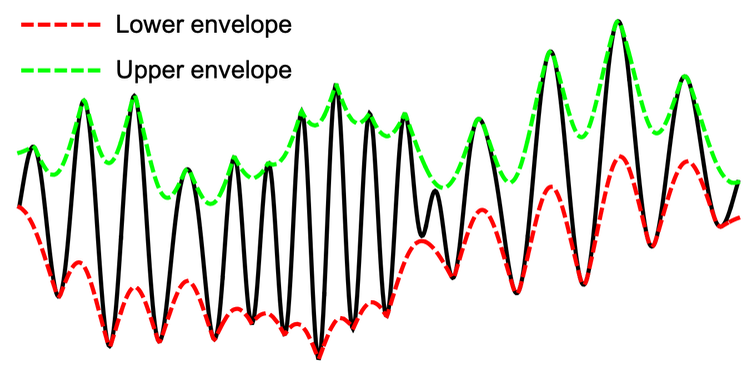✕ `CloudGet["https://wolfr.am/T6bQCgjm"]`

MFCCs were first introduced to characterize the seismic echoes that result from earthquakes. In order to obtain MFCCs, we first apply the Fourier transform over the original sound wave on the time domain, then apply the logarithm of the magnitude over the resulting spectrum, and finally apply a cosine transformation. This resulting spectrum, called the cepstrum in the quefrency domain, is neither in the frequency domain nor in the time domain.

We will use the "AudioMFCC" option with NetEncoder to make this entire process automatic. We can also choose the amount of coefficients we want in the result with the "NumberOfCoefficients" option: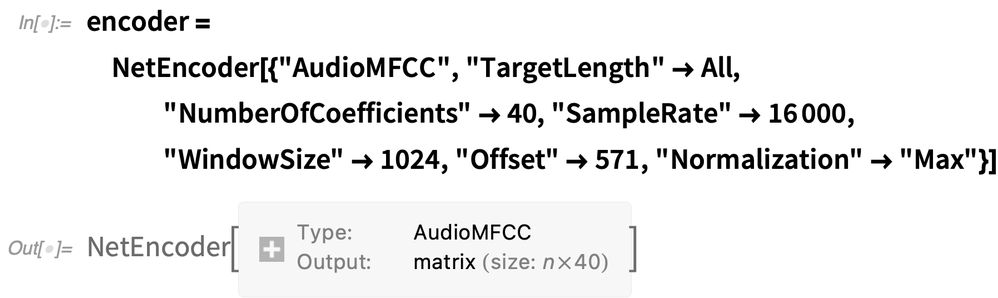✕ ```encoder = NetEncoder[{"AudioMFCC", "TargetLength" -> All, "NumberOfCoefficients" -> 40, "SampleRate" -> 16000, "WindowSize" -> 1024, "Offset" -> 571, "Normalization" -> "Max"}]```

We can check the result of "AudioMFCC" and NetEncoder applied on a random audio sample. The output of the encoder is a rank -2 tensor of dimensions {n,nc}, where n is the number of partitions after the preprocessing is applied and nc is the number of coefficients used for the computation: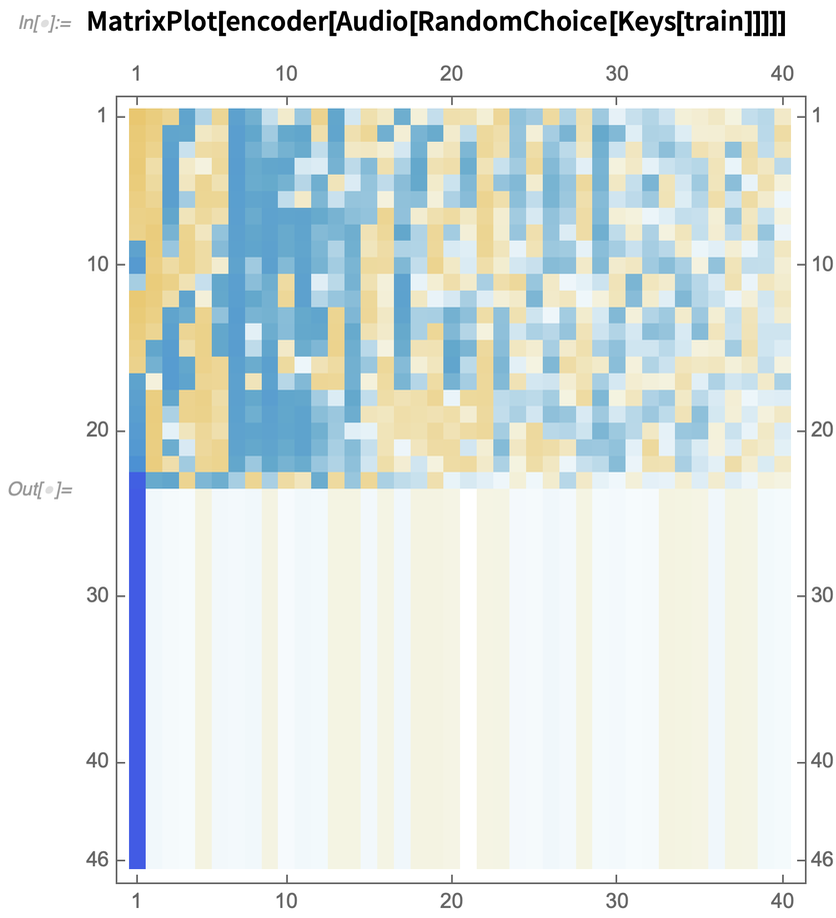✕ `MatrixPlot[encoder[Audio[RandomChoice[Keys[train]]]]]`

We can see how the audio has been converted into a matrix that represents the cepstral features of the audio. This will be the input for our model. We will build a custom recurrent neural network (RNN), for which hyperparameters have been tuned by hand, iterating them on a tune-train-evaluate process. That means the RNN will: (1) choose a set of hyperparameters; (2) train the model; (3) evaluate the model; and (4) repeat steps one through three. We repeat this process until the model shows low overfitting and high evaluation metrics. The result is the following RNN: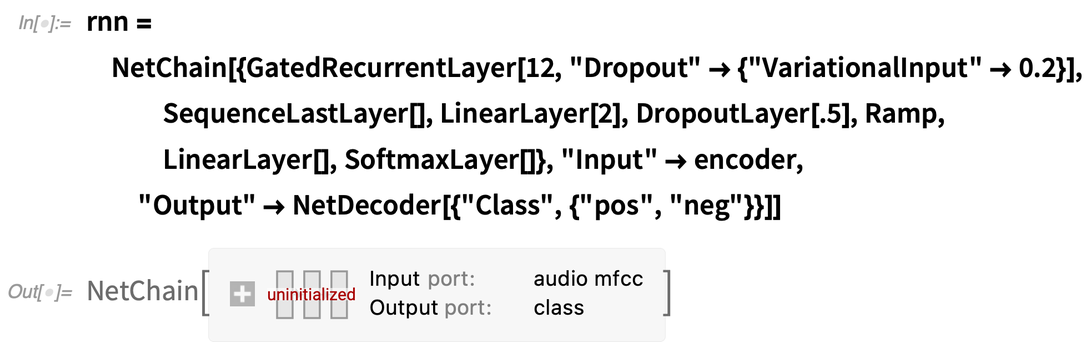✕ ```rnn = NetChain[{GatedRecurrentLayer[12, "Dropout" -> {"VariationalInput" -> 0.2}], SequenceLastLayer[], LinearLayer, DropoutLayer[.5], Ramp, LinearLayer[], SoftmaxLayer[]}, "Input" -> encoder, "Output" -> NetDecoder[{"Class", {"pos", "neg"}}]]```

We train the recurrent neural network over the training set and validate on the test set. This allows us to observe the training process and tune the hyperparameters of the network, such as the amount of neurons on the LinearLayer, the DropoutLayer number and the GatedRecurrentLayer amount of features in sequence: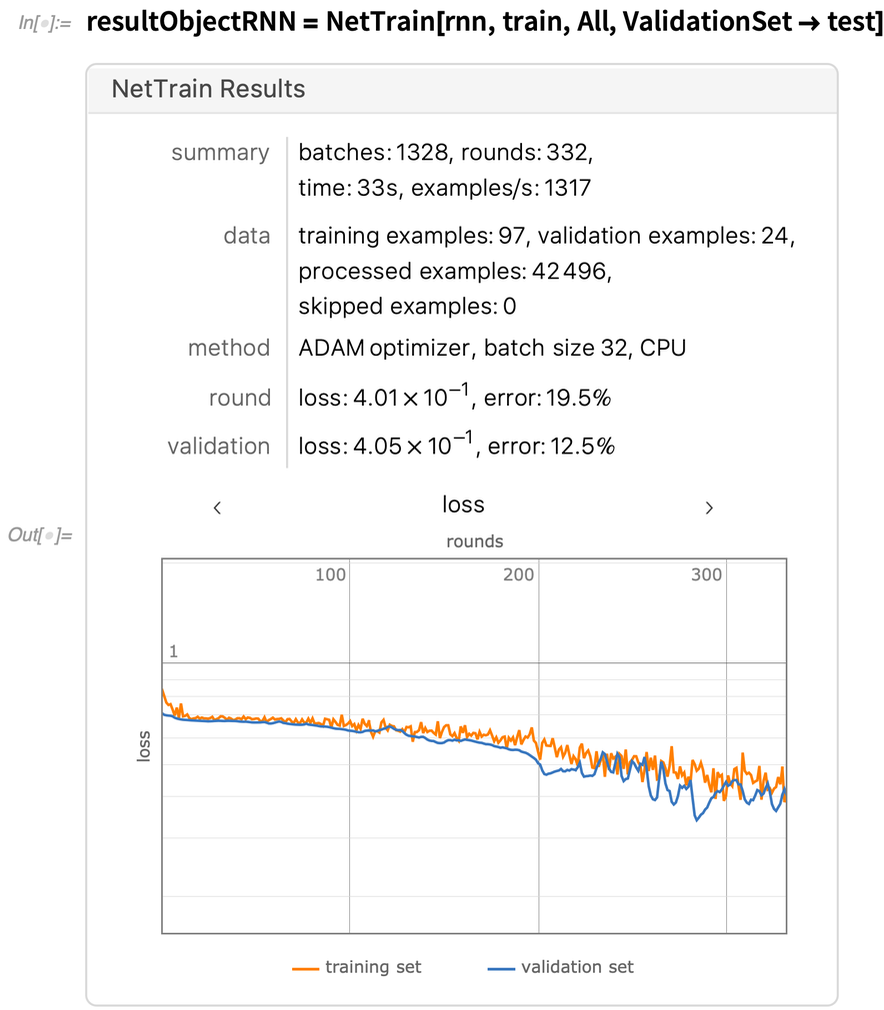✕ `resultObjectRNN = NetTrain[rnn, train, All, ValidationSet -> test]`

After the training, we will make an evaluation of the model, applying it to the previously unseen test data and measuring its performance. For that, we will try different metrics:

• Accuracy: the ratio of correctly predicted observations to the total observations.
• F1 score: the weighted average of precision and recall.
• Precision and recall: precision is the ratio of correctly predicted positive observations to the total predicted positive observations, while recall is the ratio of correctly predicted positive observations to all observations in the actual class (see an example in the following image).
• Confusion matrix plot: allows us to see the true positive, true negative, false positive and false negative predicted values.
• ROC curve: tells us how capably the model can accurately distinguish between classes (see next figure). The bigger the overlap between negative class and positive class curves, the poorer the ROC curve will be. An optimal ROC curve will be the one with an area under the curve (AUC) equal to 1.

Let’s look at the diagnostic parameters for the model: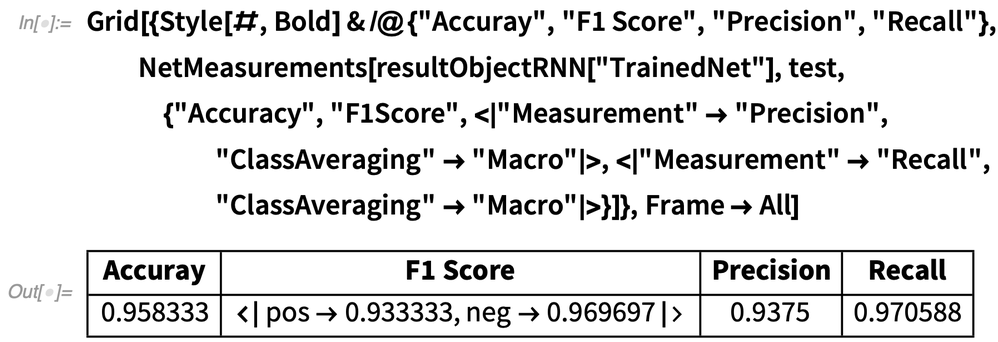✕ ```Grid[{Style[#, Bold] & /@ {"Accuray", "F1 Score", "Precision", "Recall"}, NetMeasurements[resultObjectRNN["TrainedNet"], test, {"Accuracy", "F1Score", <|"Measurement" -> "Precision", "ClassAveraging" -> "Macro"|>, <|"Measurement" -> "Recall", "ClassAveraging" -> "Macro"|>}]}, Frame -> All]```

We can also plot the confusion matrix and ROC curve of the model applied to the test set: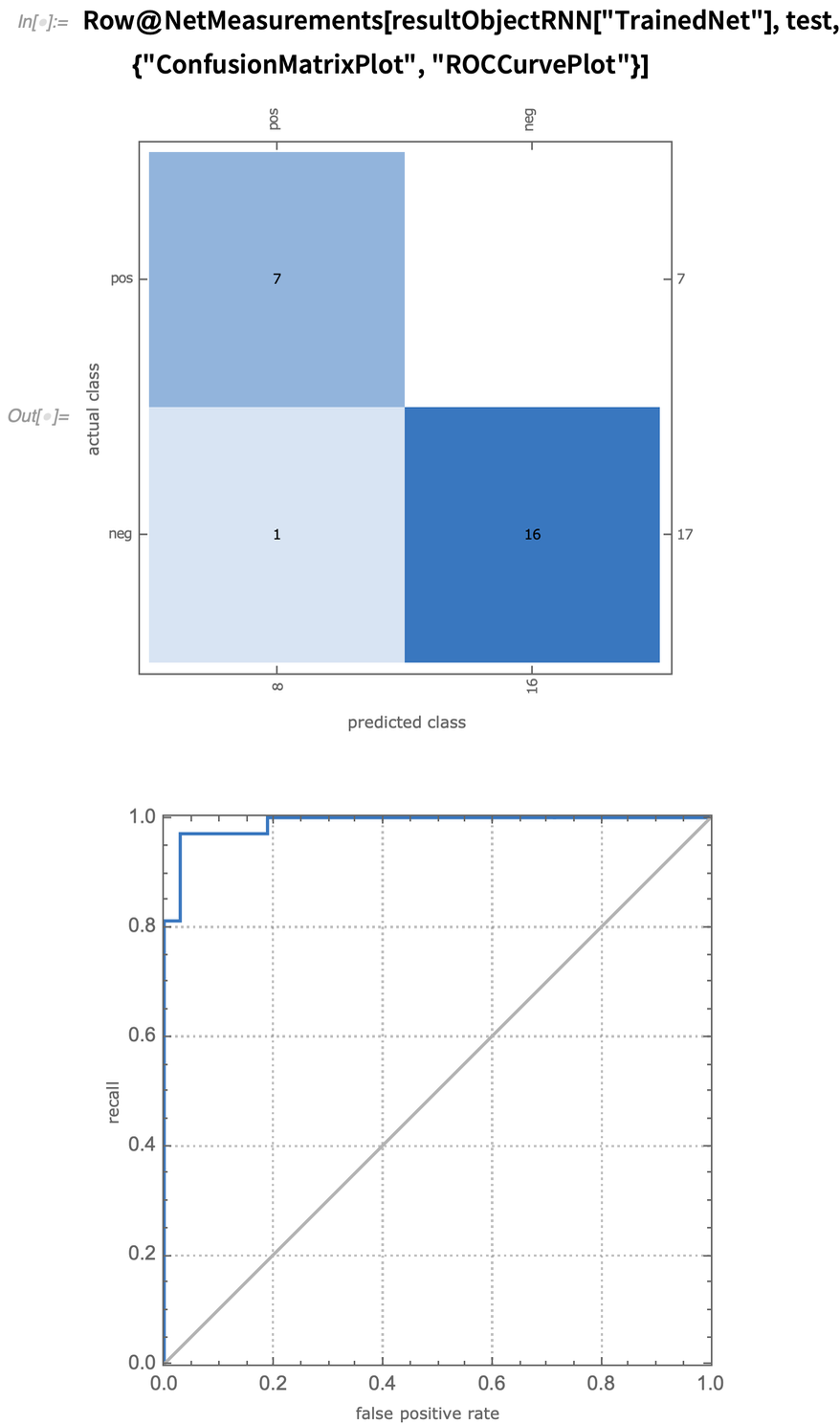✕ ```Row@NetMeasurements[resultObjectRNN["TrainedNet"], test, {"ConfusionMatrixPlot", "ROCCurvePlot"}]```

Overall, we have obtained a great performance with the metrics we have evaluated. They tell us that the model has the capacity to correctly identify or discard the presence of COVID-19 disease from patients’ cough sounds.

We have constructed a model that has the ability to detect COVID-19 by classifying cough sounds with around 96% accuracy. This shows not only the power of recurrent neural networks to solve sound classification tasks, but also the potential for solving medical tasks such as diagnosing pulmonary disease. We were able to reproduce the results published by the MIT team and the Manchester team. Our dataset was small (121 samples), but the results are promising and open the possibility of future research.

 Get full access to the latest Wolfram Language functionality with a Mathematica 12.2 or Wolfram|One trial.